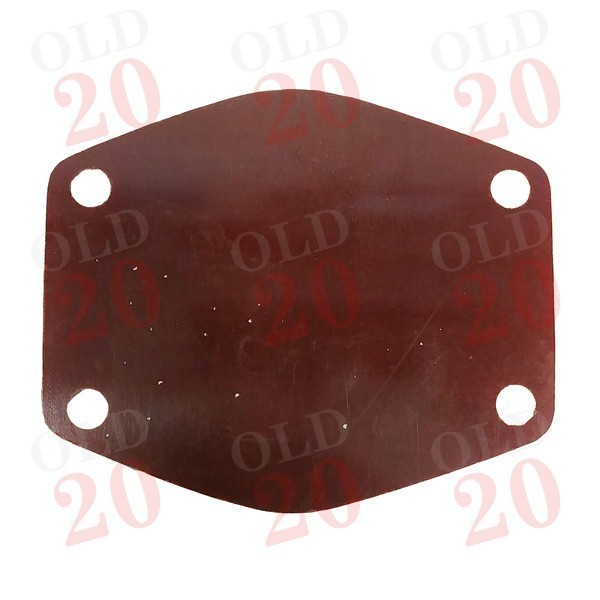View larger

1260010

New

£ 3.42Tractors < Massey Ferguson < Pre-100 Series < 35
Tractors < Massey Ferguson < Pre-100 Series < 35X
Tractors < Massey Ferguson < 100 < 133
Tractors < Massey Ferguson < 100 < 134C
Tractors < Massey Ferguson < 100 < 135
Tractors < Massey Ferguson < 100 < 140
Tractors < Massey Ferguson < 100 < 145
Tractors < Massey Ferguson < 100 < 148
Tractors < Massey Ferguson < 100 < 150
Tractors < Massey Ferguson < 100 < 152
Tractors < Massey Ferguson < 100 < 154
Tractors < Massey Ferguson < 100 < 154C
Tractors < Massey Ferguson < 200 < 230
Tractors < Massey Ferguson < 200 < 233
Tractors < Massey Ferguson < 200 < 234C
Tractors < Massey Ferguson < 200 < 235
Tractors < Massey Ferguson < 200 < 240
Tractors < Massey Ferguson < 200 < 245
Tractors < Massey Ferguson < 200 < 250
Tractors < Massey Ferguson < 200 < 253
Tractors < Massey Ferguson < 200 < 254
Tractors < Massey Ferguson < 200 < 254C
Tractors < Massey Ferguson < 200 < 255
Tractors < Massey Ferguson < 200 < 263
Tractors < Massey Ferguson < 300 < 340
Tractors < Massey Ferguson < 300 < 342
Tractors < Massey Ferguson < 300 < 350
Tractors < Massey Ferguson < 300 < 355

Supplier Reference :

 Section Cooling